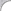Integer
Hex
Octal
Binary
Float
Scientific

## Special:

Use my meta-search engine, MultiSeek to "find" the answers to your calculations. Especially useful with the Firefox search bar plugin, available anywhere any time, just type "@calc"!

## Basic help:

Combine values and function calls into expressions with the operators, following the standard rules of arithmetic. The expressions are logged in the ticker on the left side of the screen, with the answer displayed just to the right (or below, in the case of long expressions / answers) of the expression.

On the right side of the screen is the last answer, presented in many separate formats. Click on any answer on the left to re-display all of its formats on the right, click an expression to re-run that expression. Click on any version of the answer on the right, and it will be selected, for easy copy and paste elsewhere. Press ESC any time to re-focus the input box.

### Operators:

+
Arithmetic Plus, unary plus
-
Arithmetic Subtract, unary negation
*
Arithmetic Multiply
/
Arithmetic Divide
%
Arithmetic Modulus
( )
Parentheses

### Values:

Ans, Ans0 - Ans9
Previous answer. Ans0 is the last answer, Ans1 the answer before that and so on, up to Ans9. Ans alone stands for Ans0.
-123.456
Decimal numbers. Optional leading +/- specification, optional decimal and fraction.
-1.23456E2
Decimal numbers, scientific notation. Optional leading +/- specification.
0x123
Hex numbers. Optional leading +/- specification, integers only.
0123
Octal numbers. Optional leading +/- specification, integers only.
0b1111011
Binary numbers. Optional leading +/- specification, integers only.

### Functions:

abs(x)
Absolute value of x.
acos(x), asin(x), atan(x), atan2(x), sin(x), tan(x)
If you need these, you know how to use them.
ceil(x)
The minimum integer greater than or equal to x.
clear(x)
Clear the ticker and the saved history (where available).
floor(x)
The maximum integer less than or equal to x.
log(x)
The logarithm of x.
max(x, y, z, ...)
The maximum of the passed values, one or more.
min(x, y, z, ...)
The minimum of the passed values, one or more.
pow(x, y)
The value x raised to the power y.
random()
A random value between 0 and 1, inclusive.
round(x)
The value x, rounded to the nearest integer.
sqrt(x)
Square root of x.

### Unit conversion:

Units may be converted with the special in operator. For calcExample: 1 day in seconds is 86400.

• micron
• mil
• caliber
• inch
• inches
• feet
• foot
• ft
• yard
• m
• meter
• fathom
• km
• kilometer
• mi
• mile
• league

• degree
• octant
• sextant

• s
• second
• minute
• hour
• day
• week
• year

• mililiter
• ml
• teaspoon
• tablespoon
• floz
• fluid ounce
• cup
• pint
• quart
• gallon
• liter
• barrel

• ounce
• carat
• g
• gram
• lb
• pound
• kg
• kilogram
• stone

#### Area Units

• inch3
• square inch
• square inches
• ft2
• square feet
• square foot
• yd2
• square yard
• m2
• square meter
• acre
• km2
• square kilometer
• mi2
• square mile

## Examples:

4 + 5 * 6
34
(24*60*60)/150000
0.576
3 years in weeks
156.42857142857142